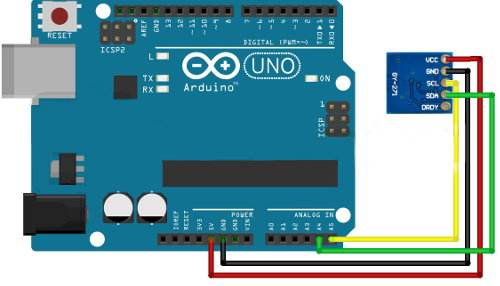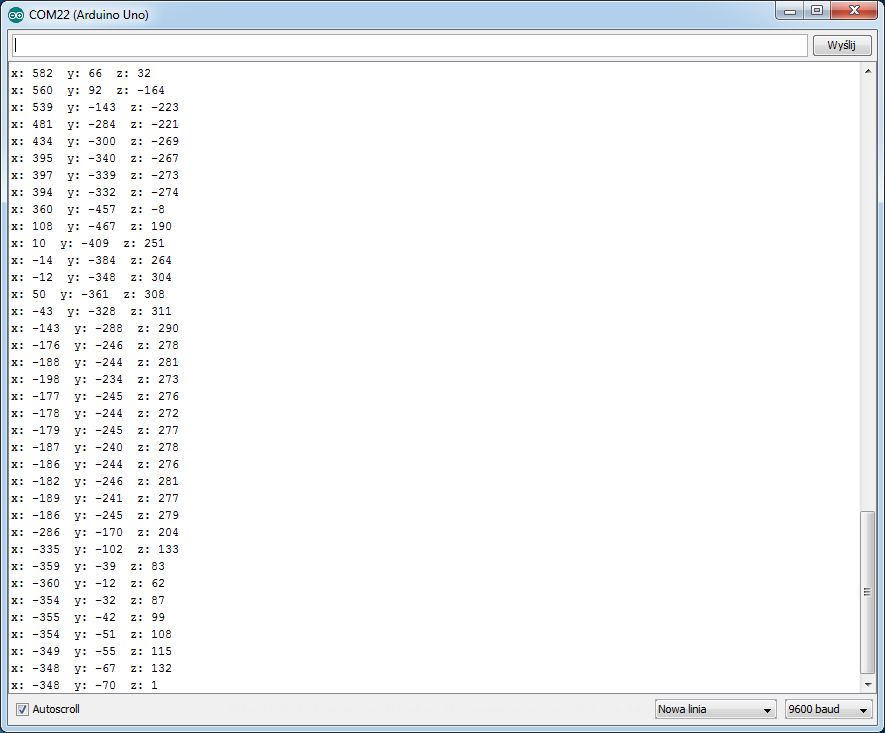# Arduino and 3-axis HMC5883L magnetometer

## 3-axis magnetometer HMC5883L and Arduino

The tutorial shows how to handle a digital magnetometer with an Arduino.

#### Connect the sensor with Arduino:

The module communicates using the popular I2C bus. Thus, the connection to the Arduino needs to be done in the following way:

Module HMC5883LPin Arduino
VCC5 V
GNDGND
SCLA5
TrafficA4Wiring diagram of the magnetometer with Arduino Uno.

#### Program for Arduino

In the example, we used the following code:

```#include  //I2C library

void setup(){
Serial.begin(9600); //initialize serial communication
Wire.begin(); //initialize the module

//Configure the appropriate sensor mode
Wire.write(0x02); //select mode register
Wire.write(0x00); //continuous measurement
Wire.endTransmission();
}

void loop(){

int x,y,z; //variables for the three axes

Wire.write(0x03); //select register 3, the register MSB of X-axis
Wire.endTransmission();

//Read data for each axis, two entries on the same axle
if(6<=Wire.available()){
x = Wire.read()<<8; //MSB of X-axis
x |= Wire.read(); //LSB X axis
with the = Wire.read()<<8; //MSB of axis
y = Wire.read()<<8; //MSB of Y-axis
y |= Wire.read(); //LSB Y axis
}

//Display data on serial monitor
Serial.print("x: ");
Serial.print(x);
Serial.print(" y: ");
Serial.print(y);
Serial.print (": ");
Serial.println ();

delay(250); //delay between measurements 250 MS
}
```

The results of the program can be seen below:A screenshot of the serial monitor.